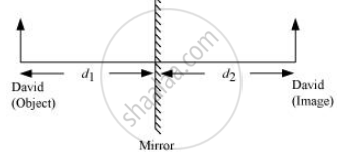Share

# David is Observing His Image in a Plane Mirror. the Distance Between the Mirror and His Image is 4 M. If He Moves 1 M Towards the Mirror, Then the Distance Between David and His Image Will Be - CBSE Class 7 - Science

ConceptReflection of Light

#### Question

David is observing his image in a plane mirror. The distance between the mirror and his image is 4 m. If he moves 1 m towards the mirror, then the distance between David and his image will be

•  3 m

•  5 m

•  6 m

• 8 m

#### Solution

6 m

In the case of a plane mirror, the distance between the object and the mirror (d1) is same as the distance between the image and the mirror (d2).Given,

Distance between the mirror and David’s image, d2 = 4 m

Therefore, d1 = d2 = 4 m

If David moves 1 m towards the mirror, then d1 = 4 − 1 = 3 m

Again, d1 = d2 = 3 m

Therefore, the distance between David and his image is d1 + d2 = 3 + 3 = 6 m

Is there an error in this question or solution?

#### APPEARS IN

Solution David is Observing His Image in a Plane Mirror. the Distance Between the Mirror and His Image is 4 M. If He Moves 1 M Towards the Mirror, Then the Distance Between David and His Image Will Be Concept: Reflection of Light.
S